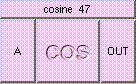COSINETHE BOX'S MAIN HELP: This function returns a scalar between [-1,1], the cosine of angle A. A (input): This must be a scalar. This is the angle in degrees. OUT (output): This function returns a scalar between [-1,1], the cosine of angle A.

<-- BACK TO Math